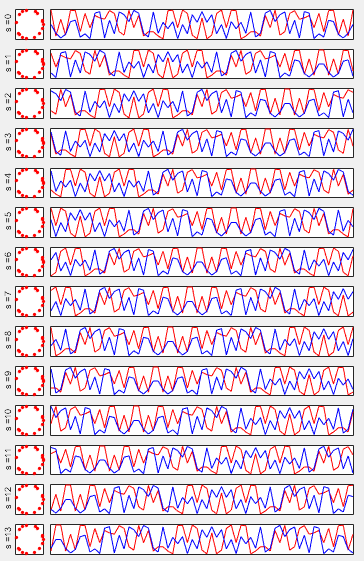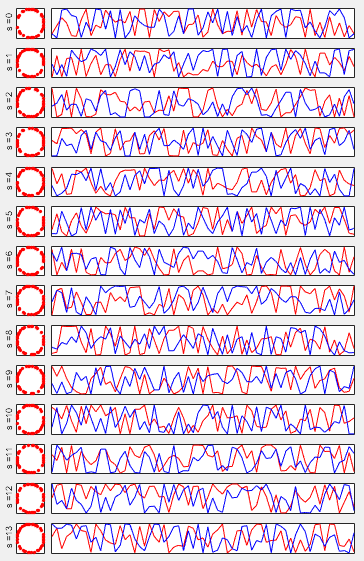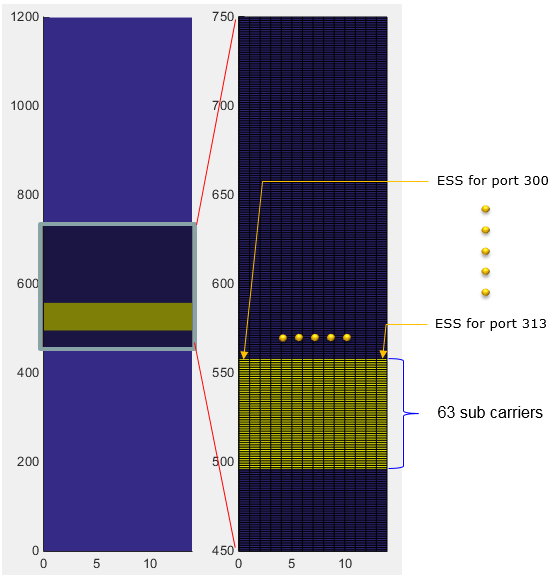5G - Pre Trial - Physical Signal - ESS                    Home : www.sharetechnote.com

ESS (Extended Synchronization Signal)

ESS is something that is not in LTE.  Main purpose of ESS is to uniquely identify the symbol number within the subframe.

• Mapped to 72 active sub carriers(6 resource blocks), centered around the DC subcarrier at every OFDM symbols in Subframe 0 and at every OFDM symbols in Subframe 25. (Note : the 5 subcarriers at the bottom and top (10 subcarriers in total) are not allocated any data, meaning only 62 subcarriers carries the real ESS data)
• The single 62 Zadoff Chu Sequence is cyclic shifted for each OFDM Symbol to give a unique identity
• The ESS sequence for each OFDM symbol is scrambled (multiplied) by a Gold Sequence which is unique for every physical cell ID.
• Used for OFDM Symbol Number Identification

Baseband Signal Generation

 Zadoff Chu Sequence for Each Symbol(Subframe 0) ESS Sequence for Each Symbol(Subframe 0)Disclaimer : This code is just to push myself (probably readers) to look into the algorithm (formula) specified in the specification to the most detailed level. If you try to convert the specification into the programming code whatever language you choose, you will understand the equation / algorithm in much more detailed level than just reading the document. However, this code has not been verified with any real data.

 Filename : Generate_Ess.m Last Update : Dec 23, 2016 %V5G.211 - 6.8.3.1 function SequenceEss = Generate_Ess(ESS)       CyclicShift = [0 7 14 18 21 25 32 34 38 41 45 52 59 61];       i = ESS.Subframe;     NID_Cell = ESS.NID_Cell;       % Generate the base Zadoff-Chu Sequence     d_n = [];       for n = 0:62         d = exp(-j*25*pi*n*(n+1)/63);         d_n = [d_n d];     end;          % Generate the Cyclic Shifted Version of the base sequence     % for each symbol     d_tilda_n = [];              for l = 0 : 13         d_tilda_n_l = [];         for n = 0:62             d = d_n(mod(n+CyclicShift(l+1),63)+1);             d_tilda_n_l = [d_tilda_n_l d];         end;         d_tilda_n = [d_tilda_n ; d_tilda_n_l];     end;          SequenceEss.d_tilda_n = d_tilda_n;          % Generate Psuedo Random Sequence          C_init = 2^10 * (i + 1) * (2 * NID_Cell + 1) + 2 * NID_Cell + 1;          r_n = [];     c_n_even = [];     c_n_odd = [];          PR.x2_init = C_init;       for n = 0 : 62       PR.n = 2*n;       c_n = Generate_PR(PR);       c_n_even = [c_n_even c_n];       PR.n = 2*n+1;       c_n = Generate_PR(PR);       c_n_odd = [c_n_odd c_n];     end;          r_n = (1 ./ sqrt(2) * (1 - 2 .* c_n_even)) + (j .* 1 ./ sqrt(2) * (1 - 2 .* c_n_odd));          % Scramble the Zadoff Chu for each symbol     d_n = [];              for l = 0 : 13         d = d_tilda_n(l+1,:) .* r_n;         d_n = [d_n; d];     end;          SequenceEss.d_n = d_n;      end

 Filename : PlotSequence_Ess.m Last Update : Dec 23, 2016 function h=PlotSequence_Ess(EssSequence,PlotOption)       if strcmp(PlotOption.PlotData,'UnScrambled') == 1        plotData = EssSequence.d_tilda_n;     end;          if strcmp(PlotOption.PlotData,'Scrambled') == 1        plotData = EssSequence.d_n;     end;          w = 10;          for l = 0:13         d_n=plotData(l+1,:);                  subplot(14,w,(l*w)+1);         plot(real(d_n),imag(d_n),'ro', ...              'MarkerFaceColor',[1 0 0],'MarkerSize',2);         set(gca,'xticklabel',[]);set(gca,'yticklabel',[]);         set(gca,'xtick',[]);set(gca,'ytick',[]);         ylabel(strcat('s = ',num2str(l)));         set(gca,'fontsize',6);           subplot(14,w,[((l*w)+2) ((l*w)+w)]);         n = 0:62;         plot(n,real(d_n),'r-',n,imag(d_n),'b-');         xlim([0 62]);         set(gca,'xticklabel',[]);set(gca,'yticklabel',[]);         set(gca,'xtick',[]);set(gca,'ytick',[]);     end   end

 Filename : Test_Generation_Ess.m Last Update : Dec 23, 2016 ESS.Subframe = 0; ESS.NID_Cell = 0;   EssSequence = Generate_Ess(ESS);   PlotOption.PlotData = 'UnScrambled'; PlotSequence_Ess(EssSequence,PlotOption);   % PlotOption.PlotData = 'Scrambled'; % PlotSequence_Ess(EssSequence,PlotOption);

RE Mapping(Resource Element Mapping) of ESSESS is a new type of Synchronization signal that is not in current LTE. The purpose of Extended synchronization Signal is to identify the OFDM symbol index and transmitted in symbol 0-13 in subframes 0 and 25. It is defined in 211-6.8.3 Extended synchronization signal.

It is made up of 62 data based on Zadoff-Chu sequence and occupy 62 subcarriers, but in resource allocation total 72 sub carrier (6 RB) is allocated for the ESS. It means 5 sub carriers on both side of ESS is reserved (not used) as a kind of gap.

The ESS is transmitted in all OFDM symbols. This is because Network in PreTrial is transmitting ESS for 14 different antenna ports as indicated below.Matlab Code :  ESS